# Further adventures with 3D Strange Attractors

After the original new 3D Strange Attractor formulas I found here I have been playing with alternate formulas and came up with these 5 new types.

Here are the formulas used and some sample images of each type. The a3da[] values are parameters between -1 and +1 that control the shapes.

Rampe 6
xnew=z*sin(a3da*x)-cos(a3da*y)
ynew=x*sin(a3da*y)+cos(a3da*z)
znew=y*sin(a3da*z)-cos(a3da*x)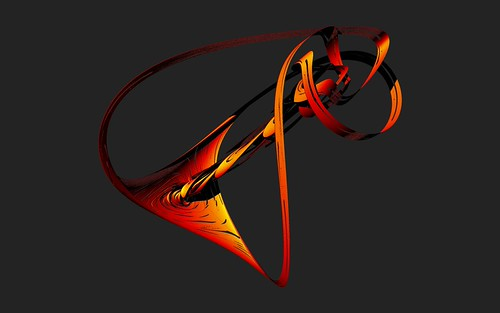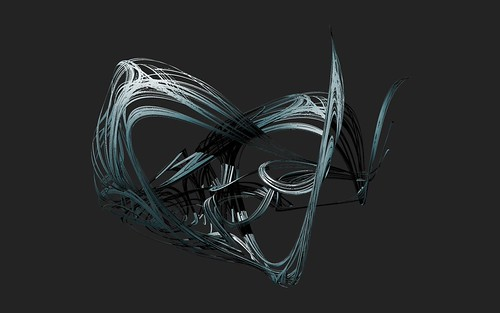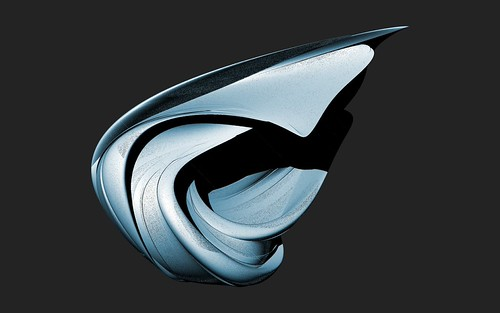Rampe 7
xnew=z*sin(a3da*x)-cos(a3da*y)
ynew=x*cos(a3da*y)+sin(a3da*z)
znew=y*sin(a3da*z)-cos(a3da*x)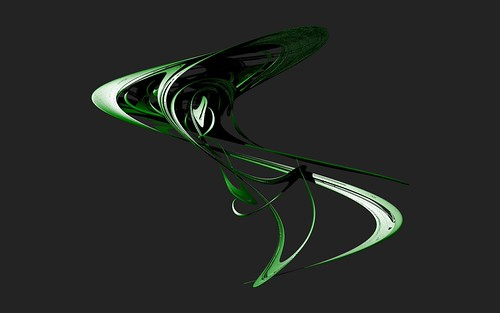Rampe 8
xnew=z*sin(a3da*x)-cos(y)
ynew=x*cos(a3da*y)+sin(z)
znew=y*sin(a3da*z)-cos(x)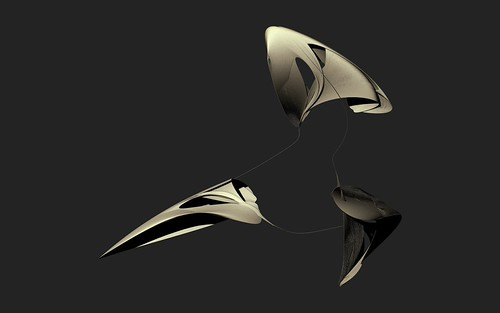Rampe 9
xnew=y*sin(a3da*x)-arccos(a3da*y)+sin(a3da*z)
ynew=z*sin(a3da*x)-arccos(a3da*y)+sin(a3da*z)
znew=x*sin(a3da*x)-arccos(a3da*y)+sin(a3da*z)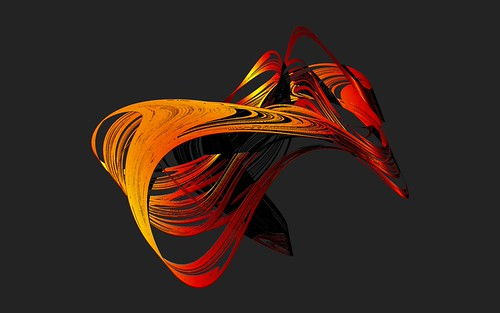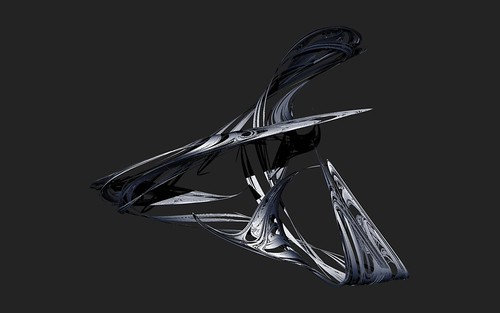Rampe 10
xnew=y*sin(a3da*x)-cos(a3da*y)+arcsin(a3da*z)
ynew=z*sin(a3da*x)-cos(a3da*y)+sin(a3da*z)
znew=x*sin(a3da*x)-cos(a3da*y)+sin(a3da*z)All of the above formulas are now implemented in the latest version of Visions Of Chaos.

I have more ideas to expand new attractor formuals in future versions of Visions Of Chaos. Firstly is to get a decent formula compiler implented so the user can try formulas easily by typing in the 3 formulas for xnew, ynew and znew. Once that works the next obvious evolution is to get it to genetically evolve and mutate the formulas like I have done for Genetic Art in the past.

Jason.

## 6 responses to “Further adventures with 3D Strange Attractors”

1.sam says:

•softologyblog says:

Not a problem.

Jason.

2.CS says:

Hello, great work. Is there a way to export these as an OBJ file or similar?

•softologyblog says:

There is no OBJ export at the moment. These images are created by calculating a “point cloud” of millions of points and then rendering them.

Would an OBJ file containing millions of points be helpful to you?

Regards,
Jason.

•CS says:

Hi Jason,
Sure that might be cool. I was hoping to import them into something like Meshlab or Blender

cheers,
CS

•softologyblog says:

OK, I added in OBJ export for the 3D Strange Attractors.

I did some tests importing the OBJ files (approx 3 million points) into both MeshLab and Blender and they worked fine. I don’t know how to create meshes or solid objects from the point clouds, so if you do get some nice results I would like to see them. I was able to use MeshLab to simplify the points, but any marching cube options I tried crashed Meshab.